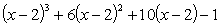§2  代数方程的性质

[代数基本定理]  每个复数域上n次代数方程

f(x)=a0xn+a1xn-1+L+an1x+an=0           (n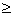1)

[多项式的导数]  多项式f(x)的导数为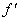(x)=na0xn1+(n1)a1xn2+L+an1

[单根与重根]

x1,x2,L,xk分别为f (x)α1,α2,L,αk(α1+α2+L+αk=n)重根，则

f (x)=a0(xx1)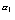(xx2)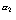L(xxk)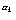[洛尔定理及其推论]  由微分学中的洛尔定理可知，在实系数方程f (x)=0的两个实根之间总有(x)=0的一个实根.

f(x)的一切根都是实的，则(x)的一切根也是实的.f(x)的相邻两根之间有(x)的一个根并且是一个单根.

f(x)的一切根都是实的，且其中有p个（计算重根）是正的，则(x)p个或

p1个正根.

[多项式的相关]

多项式f (x)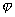(x)的根完全相同的充分必要条件是f (x)(x)只差一个不等于零的常数因子.

[整根与有理根] 任意整系数方程f (x)=0，若有一个有理根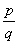（为既约分数），则pαn的约数，qα0的约数.

由此可推出：任意整系数方程的整根必为常数项的约数，若整系数方程的首项系数为1，则它的有理根必为整数.

[实根与复根，共轭实根与共轭复根]

任意有理系数方程f (x)=0，若有一个根a+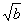(a,b是有理数，是无理数)，则必有另一个根a.这时a+a称为一对共轭实根.

任意实系数方程f (x)=0的复根只可能是成对的共轭复根，并且根的重数相同.从而，复根的个数是偶数.

任意实系数奇数次方程f (x)=0至少有一个实根.

任意实系数偶数次方程f (x)=0,a0an<0,则至少有两个实根（一个正根和一个负根）.

[根与系数的关系]

f (x)=xn+a1xn1+L+an

x1+x2+L+xn=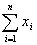=a1

x1x2+x1x3+L+xn-1xn=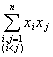=a2

x1x2x3+x1x2x4+L+xn-2xn-1xn=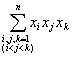=a3

LLLLLL

x1x2Lxn=(1)nan

[根的范围]  ξ为复系数代数方程

f (x)=a0xn+a1xn-1+L+an-1x+an=0                                       (1)

若所有系数ai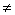0 (i=0,1,L,n),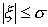,其中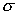为实系数代数方程

F(x)=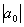xn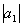xn1-L-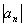=0

γ1,γ2,L,γn-1为任意正数，则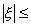τ,其中τ为下列n个数中最大的一个: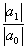+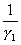,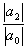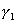+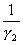,  L,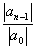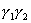L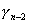+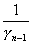,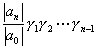max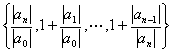(2)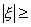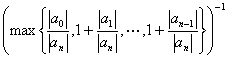(3)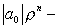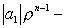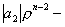L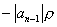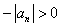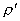>m，使得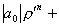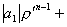L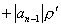γ为任意正数，则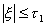，其中

τ1=max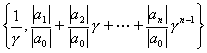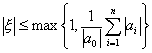若所有系数都为正实数，则

min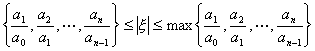若方程（1）的系数满足不等式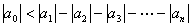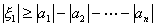[多项式的分解]

f (x)为实数域上的多项式，若有非常数的实系数多项式g(x)h(x),使得

f (x)=g(x)h(x)

实数域上不可约多项式，除一次多项式外，只有含（共轭）复根的二次多项式.

每个实系数多项式都可分解为实系数的一次因式与二次因式之积.

有理数域上的多项式的分解见第二十章，§52.

[余数定理与综合除法]  c为一常数，则多项式f (x)除以xc所得的余数等于f(c).

f (x)=a0xn+a1xn-1+L+an1x+an

f (x)除以xc的商式与余数其计算格式如下：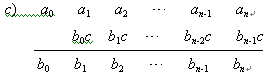f (x)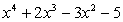除以 x2. 列出算式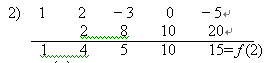[多项式的泰勒公式（秦九韶法）]  n次多项式

f (x)=a0xn+a1xn-1+L+an-1x+an         (a00)

f (x)=b0(xc)n+b1(xc)n-1+L+bn-1(xc)+bn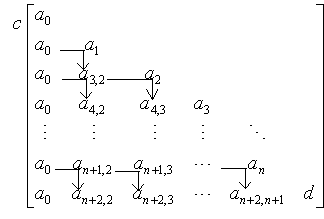ai,jc+ai,j+1=ai+1,j+1  (i=2,L,n+1; j=1,L,i1)

b0=a0,  bi=an+2,i+1    (i=1,2,L,n)

f (x)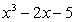x=2处的泰勒展开式.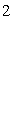f (x)